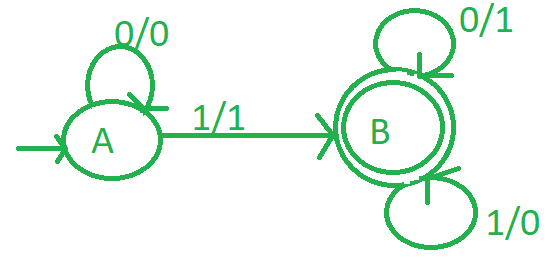# Design a mealy machine for 2’s complement

Mealy machine is a finite-state machine, its current state and the current inputs determines the output of this machine.

2’s complement :
It is the mathematical operation on binary numbers. It is used for computation as a method of signed number representation. Its complement with respect to 2N defines the two’s complement an N-bit number.

Logic:-
First calculate 1’s compliment of binary number, convert 1 to 0 and 0 to 1 and then add 1 to it. For example, if binary number is 1011 then its 1’s compliment is 0100 and its 2’s compliment is 0101

Design mealy machine :

1. Take initial state A.
2. If there are n number of zeros at initial state, it will remain at initial state.
3. Whenever first input 1 is found then it gives output 1 and go to state B.
4. In state B, if input is zero, output will be 1.And if input is 1 then output will be 0.
5. And then set state B as final state.

The approach goes as follows:

1. Start from right to left.
2. Ignore all 0’s.
3. When 1 comes ignore it and then take 1’s complement of every digit.Figure – Mealy machine of 2’s compliment

Example-1:

1. Lets take 001 and we know that its 2’s complement is (110+1 = 111).
2. So scan from right to left.
3. On state A ‘1’ came first to go to stage B and in output write 1.
4. On state B replace ‘0’ with ‘1’ and vice-versa.
5. So finally we got 111 as output.
6. Be aware that the output is also printed in right to left order.

Example-2:

1. Lets take 01 and we know that its 2’s complement is (10+1 = 11).
2. So scan from right to left.
3. On state A ‘1’ came first to go to stage B and in output write 1.
4. On state B replace ‘0’ with ‘1’ and vice-versa.
5. So finally we got 11 as output.
6. Be aware that the output is also printed in right to left order.

Don’t stop now and take your learning to the next level. Learn all the important concepts of Data Structures and Algorithms with the help of the most trusted course: DSA Self Paced. Become industry ready at a student-friendly price.

My Personal Notes arrow_drop_upCheck out this Author's contributed articles.

If you like GeeksforGeeks and would like to contribute, you can also write an article using contribute.geeksforgeeks.org or mail your article to contribute@geeksforgeeks.org. See your article appearing on the GeeksforGeeks main page and help other Geeks.

Please Improve this article if you find anything incorrect by clicking on the "Improve Article" button below.

Article Tags :

Be the First to upvote.

Please write to us at contribute@geeksforgeeks.org to report any issue with the above content.